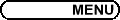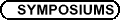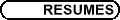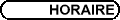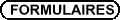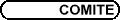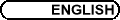Financial Mathematics / Mathématiques financières(Org: Joe Campolieti, David Vaughan, and/et Yongzeng Lai, Wilfrid Laurier University) JOE CAMPOLIETI, Wilfrid Laurier University A Path Integral Formulation for Pricing Exotic Derivatives under Alternative Price Processes A path integral formulation for pricing generally exotic derivative securities is presented. Some main features of the approach will be discussed, such as its applicability for pricing virtually any type of path dependent and early exercise option. In particular, we discuss the use of new analytically tractable pricing kernels for valuing exotics under multi-parameter "hypergeometric" (i.e. alternative) state-dependent models as well as jump processes. Moreover, we show how our approach presents a natural setting for exploiting various Monte Carlo simulations as well as lattice methods using parallel numerical algorithms for exotic option pricing. OLIVER CHEN, University of Toronto Discrete Credit Barrier Models A discrete lattice model appropriate for emulating credit rating processes-including the possibility of default-is presented. This includes a real-world process that matches historical migration probabilities and historical default probabilities, and a risk-neutral process that matches market spread rates. The processes are constructed such that the probability kernels can be expressed in terms of the Hahn polynomials: a family of orthogonal polynomials of a discrete variable, which enables numerically efficient calculation of the kernels. ALEXEY KUZNETSOV, Department of Mathematics, University of Toronto Unifying the Three Volatility Models In my talk I will introduce a method for building analytically tractable option pricing models which combine state dependent volatility, stochastic volatility and jumps. The method of eigenfunction expansion in orthogonal polynomials combined with subordination is used to add stochastic volatility and jumps to hypergeometric Brownian motions. I will also discuss how to construct analytically tractable lattice approximations for these processes, which is useful for pricing American-style options. The numerical results I will present show that such comprehensive unified models are both easy to implement and are able to reflect the complexities of the exotic option prices. GEORGE LAI, Wilfrid Laurier University Simulating Financial Derivative Sensitivities by Malliavin Calculus and Quasi-Monte Carlo Methods A lot of works have been done on Malliavin calculus since it was first developed by Paul Malliavin in the 1970s. It is a tool for analysis on Wiener space. In the past few years, Malliavin calculus was applied in finance and showed its advantages over conventional methods. But for many practitioners, and even for mathematicians, Malliavin calculus is very theoretical and rather sophisticated. The sensitivities (also called Greek letters) of financial derivatives (such as options) are very important in financial risk management. I will give an informal introduction to Malliavin calculus, and derive formulas for financial derivative sensitivities. Monte Carlo (MC) and quasi-Monte Carlo (QMC) simulation methods are applied to obtain the numerical values of sensitivities. The advantages of Malliavin calculus combined with QMC method will also be demonstrated. STEPHAN LAWI, University of Toronto Generating Functions for Stochastic Integrals Generating functions for stochastic integrals have been known in analytically closed form for just a handful of stochastic processes: namely, the Ornstein-Uhlenbeck, the Cox-Ingersoll-Ross (CIR) process and the exponential of Brownian motion. In virtue of their analytical tractability, these processes are extensively used in modeling applications. In this paper, we construct broad extensions of these process classes. We show how the known models fit into a classification scheme for diffusion processes for which generating functions for stochastic integrals and transition probability densities can be evaluated as integrals of hypergeometric functions against the spectral measure for certain self-adjoint operators. We also extend this scheme to a class of finite-state Markov processes related to hypergeometric polynomials in the discrete series of the Askey classification tree. ROMAN MAKAROV, Wilfrid Laurier University, 75 University Avenue West, Waterloo, Ontario, Canada Efficient Monte Carlo Pricing of Path Dependent Options Using High Performance Computing We study path integral algorithms for pricing discrete-time path dependent options. For underlying asset price process a nonlinear state dependent volatility model is considered. In particular, we use as new family multi-parameter volatility models, as the CEV model, for which pricing kernels are related to an underlying squared Bessel process. Efficient Monte Carlo schemes for accurate evaluation of the integrals are then presented. The algorithms combine variance reduction techniques with distributed computing to significantly increase efficiency. Moreover, we demonstrate how the Bessel bridge process can be used for sampling discrete-time paths. By rearranging variables in path integral the increment of the process can be assigned to the first sampling coordinates. Using subsequent splitting of the path integral allows us to combine bridge sampling algorithm with either adaptive Monte Carlo algorithms, or quasi-Monte Carlo techniques. Furthermore, the problem of using randomized quasi-Monte Carlo for sampling heavy-tailed distribution is investigated. The technique which combines scrambling and random shift methods is proposed. MARK REESOR, SHARCNET Research Chair in Financial Mathematics, Department of Applied Mathematics, University of Western Ontario, London, Ontario Distortion, Relative Entropy Optimisation, and Copulas Relative entropy optimisation and distortion are two methods commonly used to reweight probability distributions. The connection between these methods has recently been established and discussed in the context of risk-adjusted distributions and risk measurement (McLeish and Reesor (2002)). Copulas provide a method of constructing multivariate distributions from univariate marginals by imposing a certain dependence structure. In many cases, this can be thought of as a probability reweighting of the independent marginals to obtain a desired joint distribution. Furthermore, for a given joint distribution (copula) it can be desirable to reweight the probabilities so that the distorted joint distribution satisfies certain properties (Wang (1997), Genest and Rivest (2001), and Bennett and Kennedy (2003)). Here, we examine the connection between copulas, distortion, relative entropy optimisation, and risk.